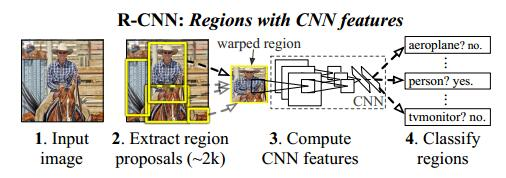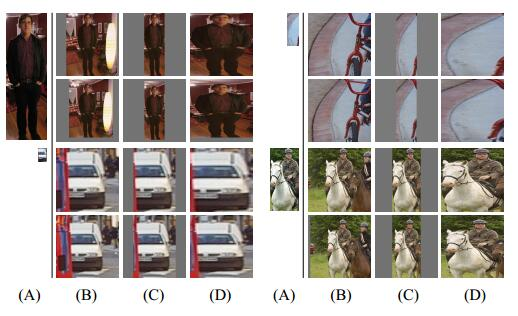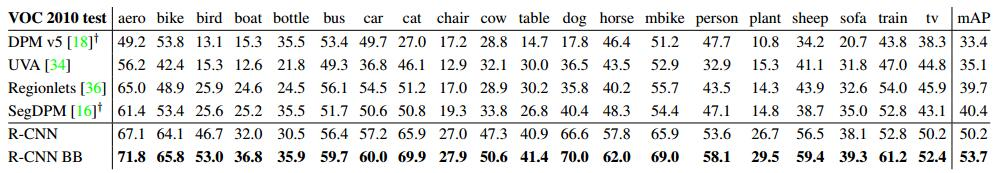0%
##### 知识点总结
• 首先利用Selective Search方法提取Region Proposal
• Region Proposal缩放后送入到CNN网络，为什么要缩放，因为fc层要求固定大小的输入。
• CNN提取特征后，送入到后面的SVM进行分类（这里还涉及到难例挖掘）
• 回归部分，利用4个线性回归，对proposal的坐标计算。
• CNN使用预训练模型，然后在VOC上进行finetune

##### 重要链接

GitHub代码链接：https://github.com/rbgirshick/rcnn

CSDN博客：http://blog.csdn.net/Chunfengyanyulove/article/details/79548472

##### 正文

R-CNN是将深度学习用于目标检测的鼻祖之作，在目标检测精度几年不在提升之后，得到了约30%的精度提升，之后，开启了深度学习在目标检测领域的一统天下，包括后面提出的Fast R-CNN、Faster R-CNN等，不仅在精度上有了进一步的提升，在速度上也有较大的进步。##### 利用Selective Search提取候选框

1. 直接使用回归进行定位，但是作者说该方法效果较差。
2. 利用sliding-window，但是由于特征提取中我们的网络较深，所以最后提取的特征感受野较大，这使得sliding-window方法受限。
3. recognition using regions

该方法通过类似Selective Search等方法，在图像中提取大量的候选框，然后对每一个候选框进行特征提取分析，找到做好的定位。与Selective Search相似的算法也比较多，作者选择Selective Search也是由于Selective Search可控性较强，而且速度还可以。

##### 输入图像处理方式

1、直接截取较大的矩形区域，然后缩放到227*227。

2、直接填充训练图像的均值，得到矩形区域，然后缩放到227*227。

3、直接缩放到227*227。

4、对上述方法，预留16个像素的边界。##### 训练SVM分类器

0.3这个阈值是作者通过grid search试出来的，{0，0.1，0.2，0.3，0.4，0.5}逐一试。

##### Bounding-box Regression

$$P_i=(P_{x}^{i},P_{y}^{i},P_{w}^{i},P_{h}^{i})$$

$$G_i=(G_{x}^{i},G_{y}^{i},G_{w}^{i},G_{h}^{i})$$

$$\hat{G}_{x} = P_wd_x(P)+P_x$$

$$\hat{G}_{y} = P_hd_y(P)+P_y$$

$$\hat{G}_{w} = P_wexp(d_w(P))$$

$$\hat{G}_{h} = P_wexp(d_h(P))$$

$w_*=argmin_{w_*}\sum(t_*^i-w_*^T\phi_5(P^i))^2+\lambda||w_*||$

$t_*$定义如下：

$$t_x=(G_x-P_x)/P_w$$

$$t_y=(G_y-P_y)/P_h$$

$$t_w=log(G_w/P_w)$$

$$t_h=log(G_h/P_h)$$

##### R-CNN精度对比##### 学习特征可视化##### Ablation studies

• 针对pool5，fc6，fc7层的分类能力对比，作者发现，其实pool5的分类能力已经很强，fc6和fc7虽然占有大量的参数，但是并没有取得实质的提升。row1-3
• 对pool5,fc6,fc7采用fune-tuning进行实验对比，作者发现，fune-tuning之后，模型的识别能力提升了近8%，fc6和fc7提升明显，但是pool5提升不明显，这样说明了pool5提取的特征具有一定的泛化性。row 4-7
• 与其他方法进行对比，优势明显。row 8-10